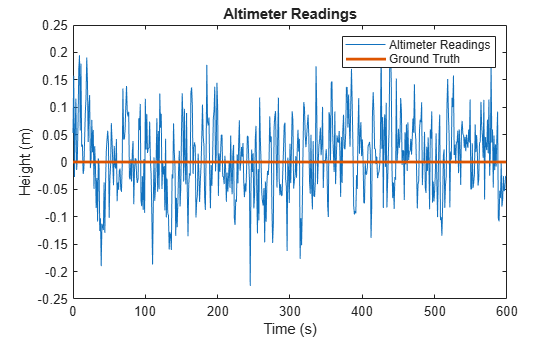# altimeterSensor

Altimeter simulation model

## Description

The `altimeterSensor` System object™ models receiving data from an altimeter sensor.

To model an altimeter:

1. Create the `altimeterSensor` object and set its properties.

2. Call the object with arguments, as if it were a function.

## Creation

### Syntax

``altimeter = altimeterSensor``
``altimeter = altimeterSensor('ReferenceFrame',RF)``
``altimeter = altimeterSensor(___,Name,Value)``

### Description

````altimeter = altimeterSensor` returns an `altimeterSensor` System object that simulates altimeter readings.```
````altimeter = altimeterSensor('ReferenceFrame',RF)` returns an `altimeterSensor` System object that simulates altimeter readings relative to the reference frame `RF`. Specify `RF` as `'NED'` (North-East-Down) or `'ENU'` (East-North-Up). The default value is `'NED'`.```
````altimeter = altimeterSensor(___,Name,Value)` sets each property `Name` to the specified `Value`. Unspecified properties have default values.```

## Properties

expand all

Unless otherwise indicated, properties are nontunable, which means you cannot change their values after calling the object. Objects lock when you call them, and the `release` function unlocks them.

If a property is tunable, you can change its value at any time.

Update rate of sensor in Hz, specified as a positive scalar.

Data Types: `single` | `double`

Constant offset bias in meters, specified as a scalar.

Tunable: Yes

Data Types: `single` | `double`

Power spectral density of sensor noise in m/√Hz, specified as a nonnegative scalar.

Tunable: Yes

Data Types: `single` | `double`

Instability of the bias offset in meters, specified as a nonnegative scalar.

Tunable: Yes

Data Types: `single` | `double`

Bias instability noise decay factor, specified as a scalar in the range [0,1]. A decay factor of `0` models the bias instability noise as a white noise process. A decay factor of `1` models the bias instability noise as a random walk process.

Tunable: Yes

Data Types: `single` | `double`

Random number source, specified as a character vector or string:

• `'Global stream'` –– Random numbers are generated using the current global random number stream.

• `'mt19937ar with seed'` –– Random numbers are generated using the mt19937ar algorithm with the seed specified by the `Seed` property.

Data Types: `char` | `string`

Initial seed of an mt19937ar random number generator algorithm, specified as a nonnegative integer scalar.

#### Dependencies

To enable this property, set `RandomStream` to `'mt19937ar with seed'`.

Data Types: `single` | `double`

## Usage

### Syntax

``altimeterReadings = altimeter(position)``

### Description

example

````altimeterReadings = altimeter(position)` generates an altimeter sensor altitude reading from the `position` input.```

### Input Arguments

expand all

Position of sensor in the local navigation coordinate system, specified as an N-by-3 matrix with elements measured in meters. N is the number of samples in the current frame.

Data Types: `single` | `double`

### Output Arguments

expand all

Altitude of sensor relative to the local navigation coordinate system in meters, returned as an N-element column vector. N is the number of samples in the current frame.

Data Types: `single` | `double`

## Object Functions

To use an object function, specify the System object as the first input argument. For example, to release system resources of a System object named `obj`, use this syntax:

`release(obj)`

expand all

 `step` Run System object algorithm `release` Release resources and allow changes to System object property values and input characteristics `reset` Reset internal states of System object

## Examples

collapse all

Create an `altimeterSensor` System object™ to model receiving altimeter sensor data. Assume a typical one Hz sample rate and a 10 minute simulation time. Set `ConstantBias` to `0.01`, `NoiseDensity` to `0.05`, `BiasInstability` to `0.05`, and `DecayFactor` to `0.5`.

```Fs = 1; duration = 60*10; numSamples = duration*Fs; altimeter = altimeterSensor('SampleRate',Fs, ... 'ConstantBias',0.01, ... 'NoiseDensity',0.05, ... 'BiasInstability',0.05, ... 'DecayFactor',0.5); truePosition = zeros(numSamples,3);```

Call `altimeter` with the specified `truePosition` to model noisy altimeter readings from a stationary platform.

`altimeterReadings = altimeter(truePosition);`

Plot the true position and the altimeter sensor readings for height.

```t = (0:(numSamples-1))/Fs; plot(t,altimeterReadings) hold on plot(t,truePosition(:,3),'LineWidth',2) hold off title('Altimeter Readings') xlabel('Time (s)') ylabel('Height (m)') legend('Altimeter Readings','Ground Truth')```